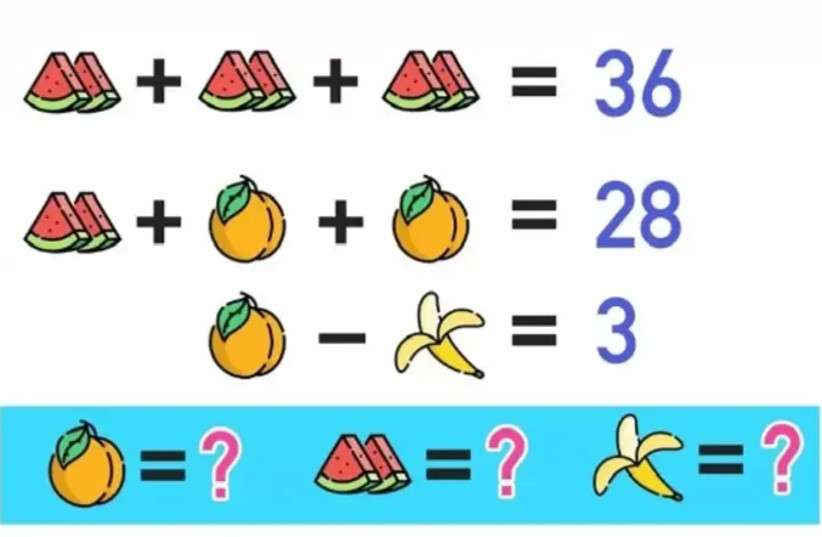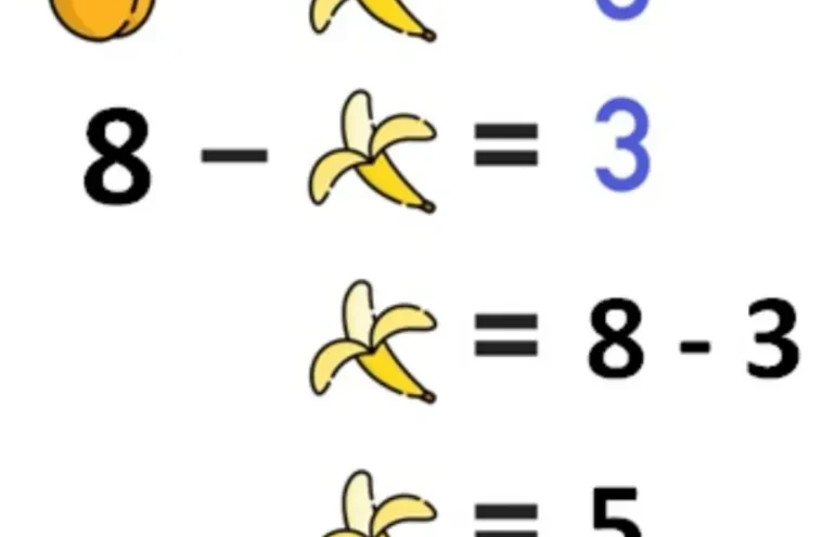(function (a, d, o, r, i, c, u, p, w, m) { m = d.getElementsByTagName(o), a[c] = a[c] || {}, a[c].trigger = a[c].trigger || function () { (a[c].trigger.arg = a[c].trigger.arg || []).push(arguments)}, a[c].on = a[c].on || function () {(a[c].on.arg = a[c].on.arg || []).push(arguments)}, a[c].off = a[c].off || function () {(a[c].off.arg = a[c].off.arg || []).push(arguments) }, w = d.createElement(o), w.id = i, w.src = r, w.async = 1, w.setAttribute(p, u), m.parentNode.insertBefore(w, m), w = null} )(window, document, "script", "https://95662602.adoric-om.com/adoric.js", "Adoric_Script", "adoric","9cc40a7455aa779b8031bd738f77ccf1", "data-key");
var domain=window.location.hostname; var params_totm = ""; (new URLSearchParams(window.location.search)).forEach(function(value, key) {if (key.startsWith('totm')) { params_totm = params_totm +"&"+key.replace('totm','')+"="+value}}); var rand=Math.floor(10*Math.random()); var script=document.createElement("script"); script.src=`https://stag-core.tfla.xyz/pre_onetag?pub_id=34&domain=\${domain}&rand=\${rand}&min_ugl=0\${params_totm}`; document.head.append(script);

# Test your intelligence: Can you solve this equation?

## The following puzzle left TikTok users confused. Will you be able to solve it?How much is each fruit worth?
(photo credit: Tiktok/Maariv)

This riddle shared on the TikTok social media platform didn't make it easy for viewers, and most weren't able to solve it. All you need to do is try to find out how much each fruit is worth in order to figure out how much all the fruits are worth together.

Here is a picture showing slices of watermelons, apricots and bananas. You need to try and figure out how much each is worth based on the information provided.

• If six watermelon slices (divided into three pairs of two slices each) together are worth 36, how much is each pair worth?
• If a pair of watermelon slices and two apricots are worth 28, how much is each apricot worth?

Look carefully at the questions and pay attention to the small details. The answer is at the bottom of this article.

### It's definitely complicated

The question was posted to TikTok with the caption: "Try to concentrate because it's definitely complicated."

Soon enough, there was a debate in the comments about the answer. If you still haven't been able to figure out the right answer, we've brought you a few different ways to help figure it out.A pair of watermelon slices is worth 12 points. (credit: Tiktok/Maariv)A banana is worth five points. (credit: Tiktok/Maariv)An apricot is worth eight points. (credit: Tiktok/Maariv)
• A pair of watermelon slices is worth 12
• An apricot is worth eight
• A banana is worth five